## Fermat's Factorization Method

Given a number, look for Integersandsuch that. Then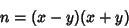(1)

andis factored. Any Odd Number can be represented in this form since then,andare Odd, and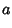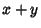(2)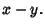(3)(4)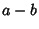(5)

so solving forandgives(6)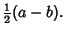(7)

Therefore,(8)

As the first trial for, try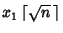, where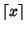is the Ceiling Function. Then check if(9)

is a Square Number. There are only 22 combinations of the last two digits which a Square Number can assume, so most combinations can be eliminated. If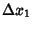is not a Square Number, then try(10)

so(11)

Continue with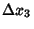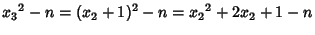(12)

so subsequent differences are obtained simply by adding two.

Maurice Kraitchik sped up the Algorithm by looking forandsatisfying(13)

i.e.,. This congruence has uninteresting solutionsand interesting solutions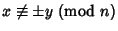. It turns out that ifis Odd and Divisible by at least two different Primes, then at least half of the solutions towith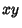Coprime toare interesting. For such solutions,is neithernor 1 and is therefore a nontrivial factor of(Pomerance 1996). This Algorithm can be used to prove primality, but is not practical. In 1931, Lehmer and Powers discovered how to search for such pairs using Continued Fractions. This method was improved by Morrison and Brillhart (1975) into the Continued Fraction Factorization Algorithm, which was the fastest Algorithm in use before the Quadratic Sieve Factorization Method was developed.

References

Lehmer, D. H. and Powers, R. E. On Factoring Large Numbers.'' Bull. Amer. Math. Soc. 37, 770-776, 1931.

Morrison, M. A. and Brillhart, J. A Method of Factoring and the Factorization of.'' Math. Comput. 29, 183-205, 1975.

Pomerance, C. A Tale of Two Sieves.'' Not. Amer. Math. Soc. 43, 1473-1485, 1996.